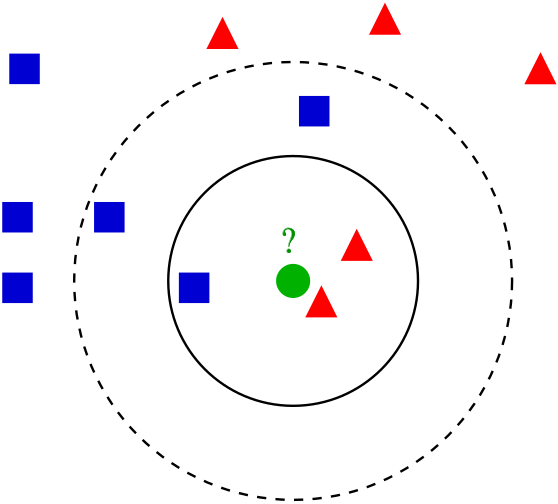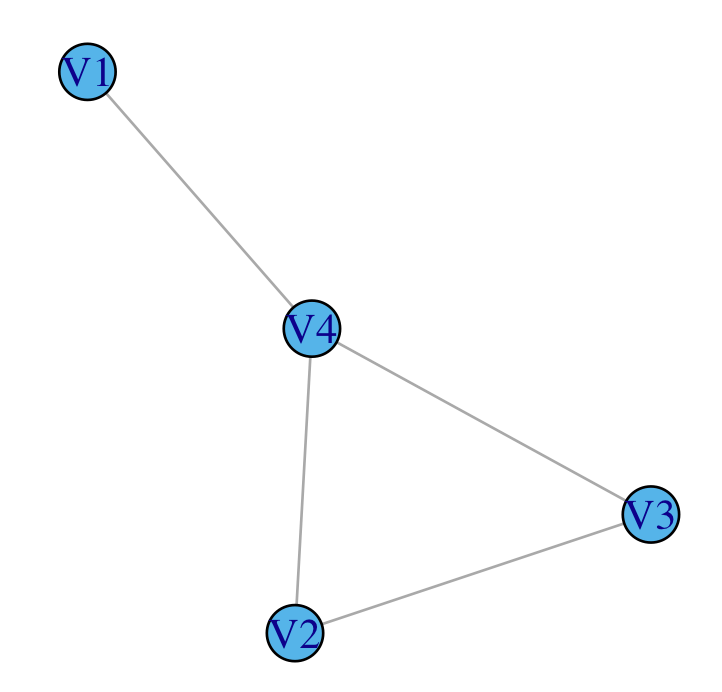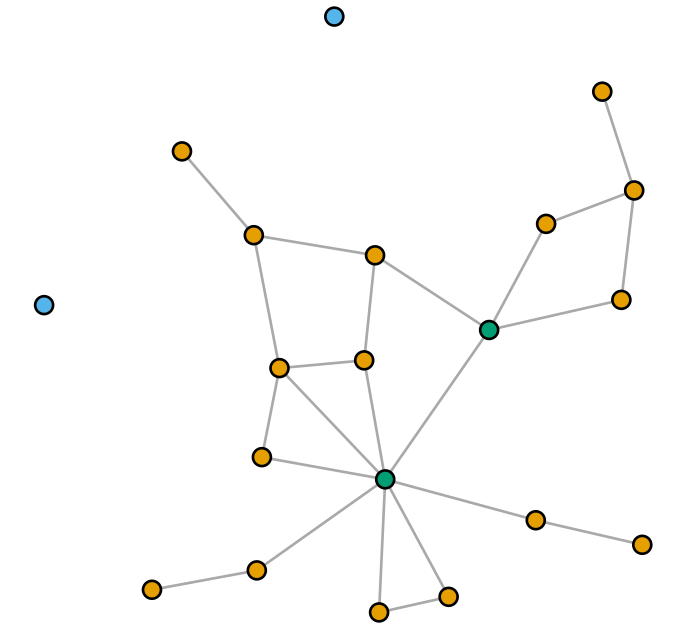# Predicting friendships and other fun machine learning tasks with graphs

Social media platforms connect users into massive graphs, with accounts as vertices and friendships as edges...

# Predicting friendships

## and other fun machine learning tasks with graphs

Noah Giansiracusa
Bentley University

Artificial intelligence (AI) breakthroughs make the news headlines with increasing frequency these days. At least for the time being, AI is synonymous with deep learning, which means machine learning based on neural networks (don't worry if you don't know what neural networks are—you're not going to need them in this post). One area of deep learning that has generated a lot of interest, and a lot of cool results, is graph neural networks (GNNs). This technique lets us feed a neural network data that naturally lives on a graph, rather than in a vector space like Euclidean space. A big reason for the popularity of this technique is that much of our modern internet-centric lives takes place in graphs. Social media platforms connect users into massive graphs, with accounts as vertices and friendships as edges (following another user corresponds to a directed edge in a directed graph), while search engines like Google view the web as a directed graph with webpages as vertices and hyperlinks as edges.A graph showing connections between people involved in the League of Nations.
Image by Martin Grandjean (CC BY-SA 3.0).

AirBnB provides an interesting additional example. At the beginning of 2021, the Chief Technology Officer at AirBnB predicted that GNNs would soon be big business for the company, and indeed just a few months ago an engineer at AirBnB explained in a blog post some of the ways they now use GNNs and their reasons for doing so. This engineer starts his post with the following birds-eye view of why graphs are important for modern data—which I'll quote here since it perfectly sets the stage for us:

Many real-world machine learning problems can be framed as graph problems. On online platforms, users often share assets (e.g. photos) and interact with each other (e.g. messages, bookings, reviews). These connections between users naturally form edges that can be used to create a graph. However, in many cases, machine learning practitioners do not leverage these connections when building machine learning models, and instead treat nodes (in this case, users) as completely independent entities. While this does simplify things, leaving out information around a node’s connections may reduce model performance by ignoring where this node is in the context of the overall graph.

In this Feature Column we're going to explore how to shoehorn this missing graph-theoretic "context" of each node back into a simple Euclidean format that is amenable to standard machine learning and statistical analysis. This is a more traditional approach to working with graph data, pre-dating GNNs. The basic idea is to cook up various metrics that transform the discrete geometry of graphs into numbers attached to each vertex. This is a fun setting to see some graph theory in action, and you don't need to know any machine learning beforehand—I'll start out with a quick gentle review of all you need.

### Machine learning

The three main tasks in machine learning are regression, classification, and clustering.

For regression, you have a collection of variables called features and one additional variable, necessarily numerical (meaning $\mathbb{R}$-valued) called the target variable; by considering the training data where the values of both the features and target are known, you fit a model that attempts to predict the value of the target on actual data where the features but not the target are known. For instance, predicting a college student's income after graduation based on their GPA and the college they are attending is a regression task. Suppose all the features are numerical—for instance, we could represent each college with its US News ranking (ignore for a moment how problematic those rankings are). Then a common approach is linear regression, which is when you find a hyperplane in the Euclidean space coordinatized by the features and the target that best fits the training data (i.e., minimizes the "vertical" distance from the training points down to the hyperplane).

Classification is very similar; the only difference is that the target variable is categorical rather than numerical—which in math terms just means that it takes values in a finite set rather than in $\mathbb{R}$. When this target set has size two (which is often $\{\mathrm{True}, \mathrm{False}\}$, or $\{\mathrm{yes}, \mathrm{no}\}$, or $\{0, 1\}$) this is called binary classification. For instance, predicting which students will be employed within a year of graduation can be framed as a binary classification task.

Clustering is slightly different because there is no target, only features, and you'd like to partition your data into a small number of subsets in some natural way based on these features. There is no right or wrong answer here—clustering tends to be more of an exploratory activity. For example, you could try clustering college students based on their GPA, SAT score, financial aid amount, number of honors classes taken, and number of intramural sports played, then see if the clusters have human-interpretable descriptions that might be helpful in understanding how students divide into cohorts.

I want to provide you with the details of one regression and classification method, to give you something concrete to have in mind when we turn to graphs. Let's do the k-Nearest Neighbors (k-NN) algorithm. This algorithm is a bit funny because it doesn't actually fit a model to training data in the usual sense—to predict the value of the target variable for each new data point, the algorithm looks directly back at the training data and makes a calculation based on it. Start by fixing an integer $k \ge 1$ (smaller values of k provide a localized, granular look at the data, whereas larger values provide a smoothed, aggregate view). Given a data point P with known feature values but unknown target value, the algorithm first finds the k nearest training points $Q_1, \ldots, Q_k$—meaning the training points $Q_i$ whose distance in the Euclidean space of feature values to P is smallest. Then if the task is regression, the predicted target value for P is the average of the target values of $Q_1, \ldots, Q_k$, whereas if the task is classification then the classes of these $Q_i$ are treated as votes and the predicted class for $P$ is whichever class receives the most votes. (Needless to say, there are plenty of variants such as weighting the average/vote by distance to P, changing the average from a mean to a median, or changing the metric from Euclidean to something else.)A green dot and its three and five nearest neighbors.
Image by Antti Ajanki (CC BY-SA 2.5).

That's all you need to know about machine learning in the usual Euclidean setting where data points live in $\mathbb{R}^n$. Before turning to data points that live in a graph, we need to discuss some graph theory.

### Graph theory

First, some terminology. Two vertices in a graph are neighbors if they are connected by an edge. Two edges are adjacent if they have a vertex in common. A path is a sequence of adjacent edges. The distance between two vertices is the length of the shortest path between them, where length here just means the number of edges in the path.

A useful example to keep in mind is a social media platform like Facebook, where the vertices represent users and the edges represent "friendship" between them. (This is an undirected graph; platforms like Twitter and Instagram in which accounts follow each other asymmetrically form directed graphs. Everything in this article could be done for directed graphs with minor modification, but I'll stick to the undirected case for simplicity.) In this example, your neighbors are your Facebook friends, and a user has distance 2 from you if you're not friends but you have a friend in common. The closed ball of radius 6 centered at you (that is, all accounts of distance at most 6 from you) consists of all Facebook users you can reach through at most 6 degrees of separation on the platform.

Next, we need some ways of quantifying the structural role vertices play in a graph. There are a bunch of these, many of which capture various notions of centrality in the graph; I'll just provide a few here.

Starting with the simplest, we have the degree of a vertex, which in a graph without loops or multiple edges is just the number of neighbors. In Facebook, your degree is your number of friends. (In a directed graph the degree splits as the sum of the in-degree and the out-degree, which on Twitter count the number of followers and the number of accounts followed.)

The closeness of a vertex captures whether it lies near the center or the periphery of the graph. It is defined as the reciprocal of the sum of distances between this vertex and each other vertex in the graph. A vertex near the center will have a relatively modest distance to the other vertices, whereas a more peripheral vertex will have a modest distance to some vertices but a large distance to the vertices on the “opposite” side of the graph. This means that the sum of distances for a central vertex is smaller than the sum of distances for a peripheral vertex; reciprocating this sum flips this around so that the closeness score is greater for a central vertex than a peripheral vertex.

The betweenness of a vertex, roughly speaking, captures centrality in terms of the number of paths in the graph that pass through the vertex. More precisely, it is the sum over all pairs of other vertices in the graph of the fraction of shortest paths between the pair of vertices that pass through the vertex in question. That's a mouthful, so let's unpack it with a couple simple examples. Consider the following two graphs:

(a)(b)In graph (a), the betweenness of V1 is 0 because no shortest paths between the remaining vertices pass through V1. The same is true of V2 and V3. The betweenness of V4, however, is 2: between V1 and V2 there is a unique shortest path and it passes through V4, and similarly between V1 and V3 there is a unique shortest path and it also passes through V4. For the graph in (b), by symmetry it suffices to compute the betweenness of a single vertex. The betweenness of V1 is 0.5, because between V2 and V3 there are 2 shortest paths, exactly one of which passes through V1.

The following figure shows a randomly generated graph on 20 vertices where in (a) the size of each vertex corresponds to its closeness score and in (b) it corresponds to the betweenness score. Note that the closeness does instead reflect how central versus peripheral the vertices are; the betweenness is harder to directly interpret, but roughly speaking it helps identify important bridges in the graph.

(a)(b)Another useful pair of measures of vertex importance/centrality in a graph are the eigenvector centrality score and the PageRank score. I'll leave it to an interested reader to look these up. They both have nice interpretations in terms of eigenvectors related to the adjacency matrix and in terms of random walks on the graph.

### Machine learning on a graph

Suppose we have data in the usual form for machine learning—so there are features for clustering, or if one is doing regression/classification then there is additionally a target variable—but suppose in addition that the data points form the vertices of a graph. An easy yet remarkably effective way to incorporate this graph structure (that is, to not ignore where each vertex is "in the context of the overall graph," in the words of the AirBnB engineer) is simply to append a few additional features given by the vertex metrics discussed earlier: degree, closeness, betweenness, eigenvector centrality, PageRank (and there are plenty others beyond these as well).

For instance, one could perform clustering in this manner, and this would cluster the vertices based on both their graph-theoretic properties as well as the original non-graph-theoretic feature values. Concretely, if one added closeness as a single additional graph-theoretic feature, then the resulting clustering is more likely to put peripheral vertices together in the same clusters and it is more likely to put vertices near the center of the graph together in the same clusters.

The following figure shows the same 20-vertex random graph pictured earlier, now with vertices colored by a clustering algorithm (k-means, for $k=3$) that uses two graph-theoretic features: closeness and betweenness. We obtain one cluster comprising the two isolated vertices, one cluster comprising the two very central vertices, and one cluster comprising everything else.If one is predicting the starting income of college students upon graduation, one could use a regression method with traditional features as discussed above but include additional features such as the eigenvector centrality of each student in the network formed by connecting students whenever they took at least one class together.

### Predicting edges

So far we've augmented traditional machine learning tasks by incorporating graph-theoretic features. Our last topic is a machine learning task without counterpart in the traditional non-graph-theoretic world: edge prediction. Given a graph (possibly with a collection of feature values for each vertex), we'd like to predict which edge is most likely to form next, when the graph is considered as a somewhat dynamic process in which the vertex set is held constant but the edges form over time. In the context of Facebook, this is predicting which two users who are not yet Facebook friends are most likely to become ones—and once Facebook makes this prediction, it can use it as a suggestion. We don't know the method Facebook actually uses for this (my guess is that it at least involves GNNs), but I can explain a very natural approach that is widely used in the data science community.

We first need one additional background ingredient from machine learning. Rather than directly predicting the class of a data point, most classifiers first compute the propensity scores, which up to normalization are essentially the estimated probability of each class—then the predicted class is whichever class has the highest propensity score. For example, in k-NN I said the prediction is given by counting the number of neighbors in each class and taking the most prevalent class; these class counts are the propensities scores for k-NN classification. Concretely, for 10-NN if a data point has 5 red neighbors and 3 green neighbors and 2 blue neighbors, then the propensity scores are 0.5 for red, 0.3 for green, and 0.2 for blue (and of course the prediction itself is then red). For binary classification one usually just reports a single propensity score between 0 and 1, since the propensity score for the other class is just the complementary probability.

Returning to the edge prediction task, consider a graph with n vertices and imagine a matrix with n choose 2 rows indexed by the pairs of vertices in the graph. The columns for this matrix are features associated to pairs of vertices—which could be something like the mean (or min, or max) of the closeness (or betweenness, or eigenvector centrality, or...) score for the two vertices in the pair, and if there are non-graph-theoretic features associated with the vertices one could also draw from these, and one could also use the distance between the two vertices in the pair as a feature. Create an additional column, playing the role of the target variable, that is a 1 if the vertex pair are neighbors (that is, joined by an edge) and a 0 otherwise. Train a binary classifier on this data, and the vertex pair with the highest propensity score among those that are not neighbors is the pair most inclined to become neighbors—that is, this is the next edge most likely to form, based on the features used. This reveals the edges that don't exist yet seem like they should, based on the structure of the graph (and the extrinsic non-graph data, if one also uses that).

If one has snapshots of the graph's evolution across time, one can train this binary classifier on the graph at time $t$ then compare the predicted edges to the actual edges that exist at some later time $t' > t$, to get a sense of how accurate these edge predictions are.

### Conclusion

In broad outlines, here's the path we took in this article and where we ended up. The distance between vertices in a graph—generalizing the popular "degrees of separation" games played with Kevin Bacon's movie roles and Paul Erdős' collaborations—allows one to quantify various graph-theoretic roles the vertices play, via notions like betweenness and closeness. These quantifications can then serve as features in clustering, regression, and classification tasks, which helps the machine learning algorithms involved incorporate the graph structure on the data points. By considering vertex pairs as data points and using the average closeness, betweenness, etc., across each pair (and/or the distance between the pair), we can predict which missing edges "should" exist in the graph. When the graph is a social media network, these missing edges can be framed as algorithmic friend/follower suggestions.

And when the graph is of mathematical collaborations (mathematicians as vertices and edges joining pairs that have co-authored a paper together), this can suggest to you who your next collaborator should be: just find the mathematician you haven't published with yet whose propensity score is highest!